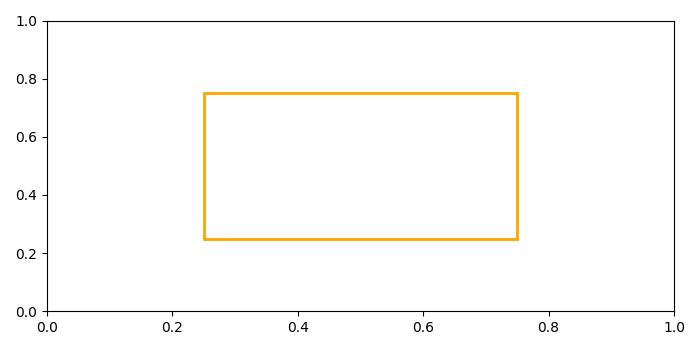# Drawing a rectangle with only border in Matplotlib

To draw a rectangle with only border in matplotlib, we can take the following steps−

• Create a figure and a set of subplots.
• Get the current axes, creating one if necessary.
• Add a patch, i.e., a rectangle to the current axes that is returned in step 2. Set the facecolor attribute to 'none'.
• To display the figure, use show() method.

## Example

from matplotlib import pyplot as plt, patches
plt.rcParams["figure.figsize"] = [7.00, 3.50]
plt.rcParams["figure.autolayout"] = True
figure, _ = plt.subplots()
ax = plt.gca()
plt.show()